# How to Square a Number in Excel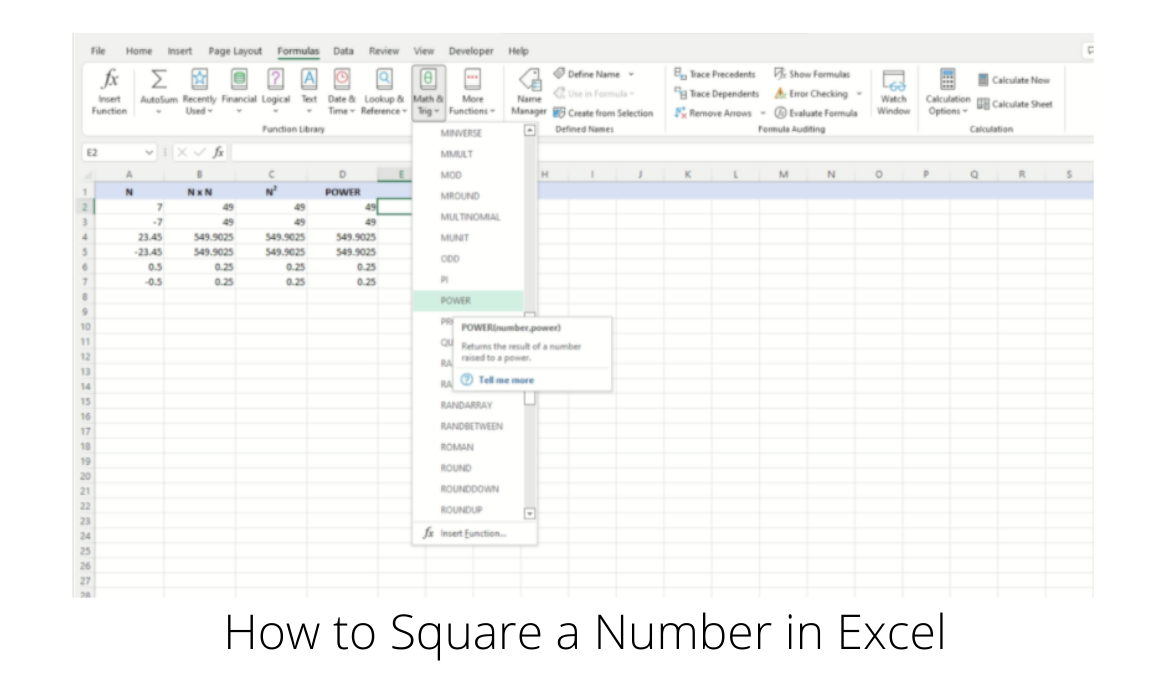Spreadsheets, in many instances, comprise large datasets, on which among other things, is usually performed mathematical operations designed to produce results that can then tell us something about the data.

An accounting based spreadsheet will total up revenues and expenses, and produce a financial statement that will indicate if a business is making a profit or loss.

A spreadsheet with temperature and precipitation measurements taken over months, can provide a statistical analysis of weather trends.

Spreadsheet applications like Microsoft Excel, are equipped with a full array of mathematical functions, as well as allowing us to create formulas of our own to help perform quite complex computations.

While we won’t be solving the mysteries of the universe in this article, we will be taking a look at a fairly simple, yet common mathematical operation, that of the square of a number.

If we have the number n, the square of n is n x n, or as it is more commonly expressed, n2 (n squared).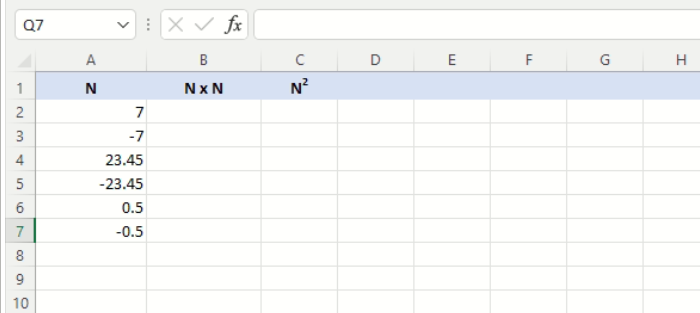We are going to be working with the above, small dataset, which contains both integer and decimal numbers for which we want to calculate the squares.

Notice also, that there are both positive and negative numbers.

Regardless of whether a number is positive or negative, squaring that number will always yield a positive result.

The first method we are going to use will reference the contents of each of the cells containing the six numbers, and calculate their square using only multiplication.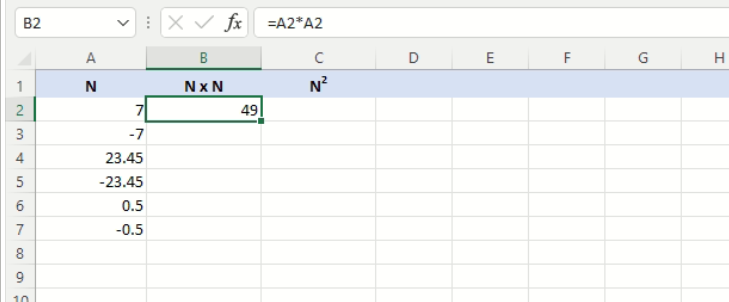To achieve this, we enter the formula =A2*A2 in cell B2, which multiplies the contents of cell A2 by itself.

As we can see above, the result produced is 49, where the contents of cell A2 is 7.

We can now apply the formula to the remaining cells.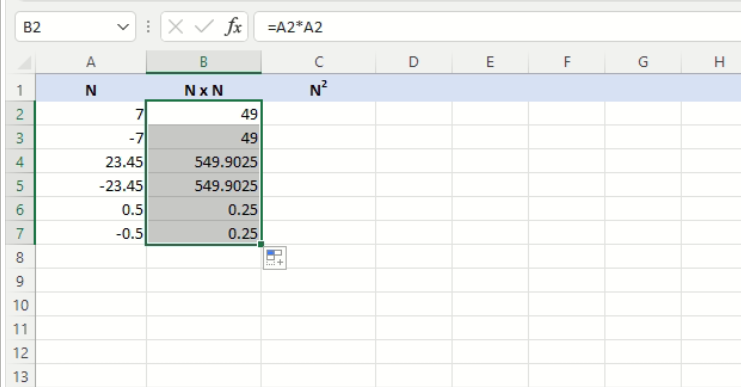To do so, when cell B2 is selected, there will be a fill handle in the bottom right hand corner of the bounding box highlighting the cell.

Grab this with the mouse, and drag it down to cell B7, thus covering the remaining cells.

This will propagate the formula to those cells, adjusting the cell reference automatically.

And as we can see, column B now has the square for each of the numbers in column A.

As was stated earlier, the square of a number is also represented as n2 (n squared or n to the power of 2).

Excel allows us to perform this operation, i.e. raise a number to a power, by using the caret ^ symbol.

Thus, we can express 7 squared, as 7^2, which represents 7 raised to the power of 2.We can now use this new expression in our sheet.

The formula becomes =A2^2, and we can apply it to cell C2.

Then, drag the fill handle to propagate the formula to the other cells in column C, as seen in the snapshot above.

We can also see that the outcome in both Columns B and C are the same, both expressions produce exactly the same results.

Excel also offers a function that allows us to raise a number to any power.

The function is aptly called POWER, and its definitions is given below.

## POWER function

Returns the result of a number raised to a power.

POWER(4,0.5)

POWER(A2,B2)

POWER(2,5)

### Syntax

POWER(number, power)

The POWER function syntax has the following arguments:

• Number    Required. The base number. It can be any real number.
• Power    Required. The exponent to which the base number is raised.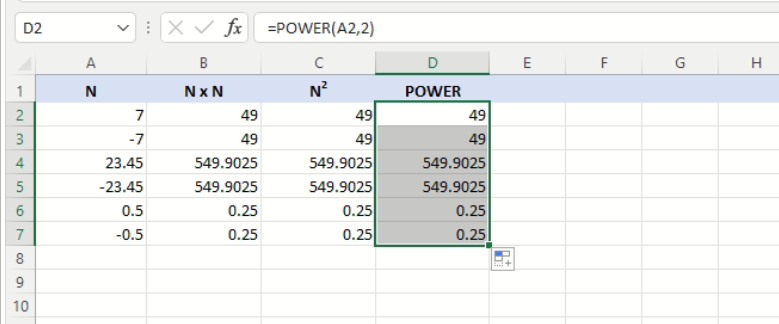Our new formula is now =POWER(A2,2), and we apply it to cell D2 as shown above, then drag the fill handle to propagate through to cell D7.

And as expected, the results are exactly the same as with those produced in columns B and C.We mentioned at the outset, that Excel is equipped with a full array of mathematical functions, and POWER is one of them.

These functions are available from the top navigation menu under Formulas > Math & Trig.

There are quite a few and you’ll need to scroll down to find the POWER function.For our example, we’ve first selected cell E2 and then selected the POWER function from the Formulas > Math & Trig menu.

This will bring up the Function Arguments dialog box shown above.

There are two input fields.

The first for the base number, and the second for the exponent or power.

You can enter plain numbers or cell references (that contain numeric values) in either field.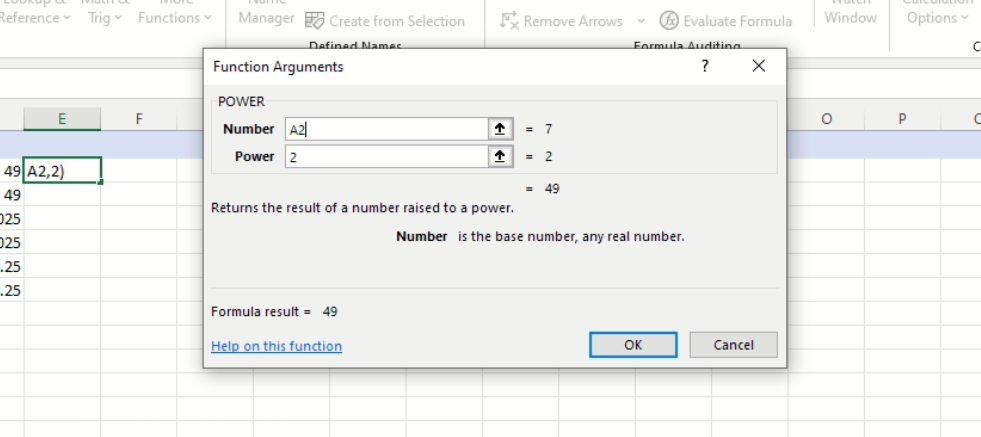In the screenshot above, just as when we used the POWER function previously, we’ve entered the cell reference A2 in the Number field, and 2 in the Power field.

Notice how the contents of cell E2 are automatically updated as we enter our values or cell references into the Number and Power fields in the dialog box.

Also notice that the result is displayed in the bottom left of the dialog box, in the Formula result = read only field.

This allows us to check the result and make adjustments before applying the formula.

Once satisfied with our values, we can click the OK button.This will generate the POWER function call in cell E2, which turns out to be exactly the same as the one we manually entered in cell D2 earlier.

Hence, entering the formula directly into the cell or invoking the Function Arguments dialog box after selecting POWER from the Formulas > Math & Trig menu, produces exactly the same outcome.

## Conclusion

Excel makes it very easy to perform a mathematical operation, such as finding the square of a number.

We also saw that as so often happens, there are a few different ways to do this and it depends on personal preference as to which method works best.

We also saw that Excel comes equipped with quite a large array of mathematical functions, and if we can’t remember the function names, we can always use the Formulas > Math & Trig menu.

Costas has a wide range of experience in Information Technology covering computer hardware, programming, telecommunications, networking, web services, and general IT support. He's worked in various roles such as PHP programmer and web developer, technical and desktop support, hardware repair, system administration. Costas has excellent background in Microsoft Windows and Office Suite (Excel, Word, Powerpoint, etc), as well as a thorough understanding of Networking and Hardware maintenance.